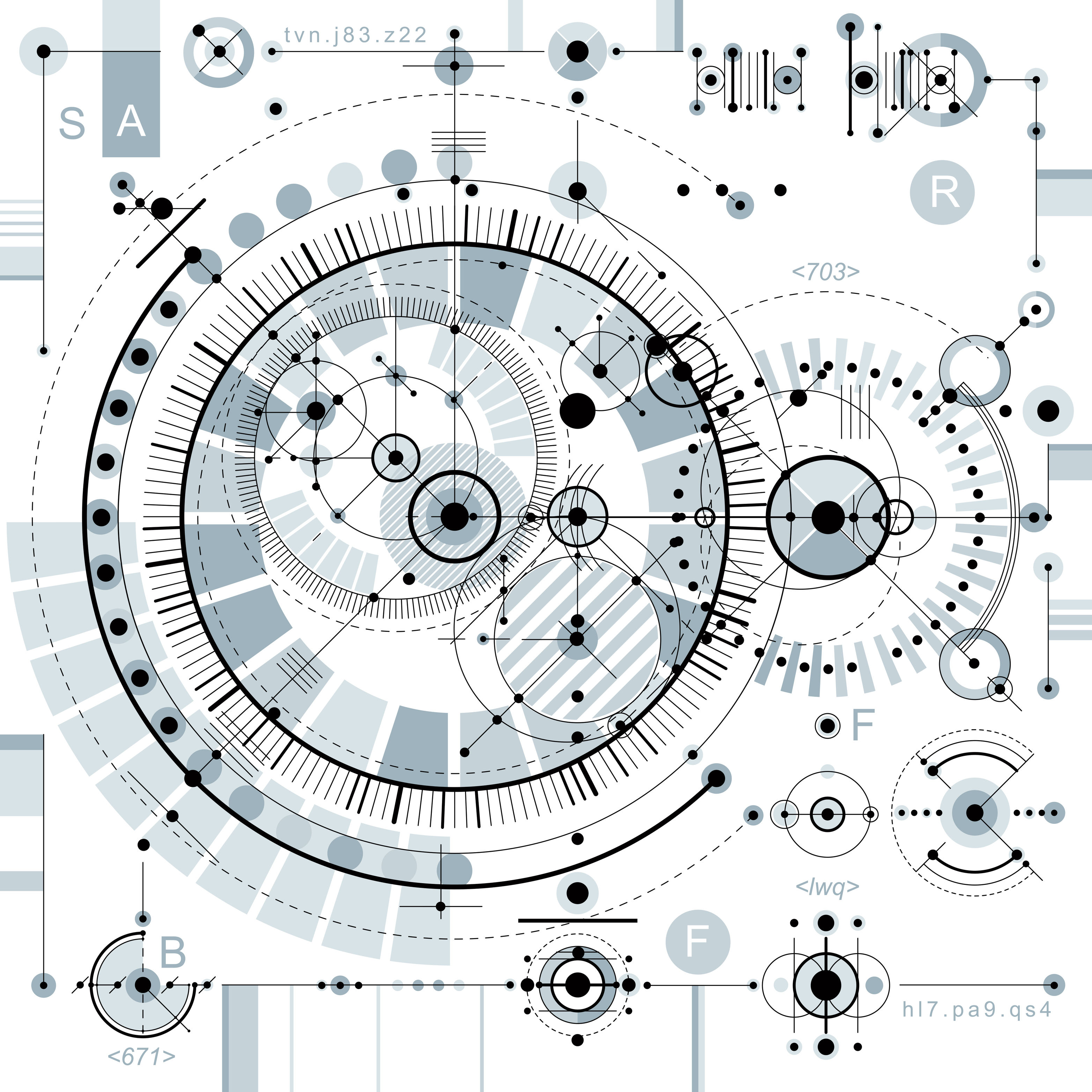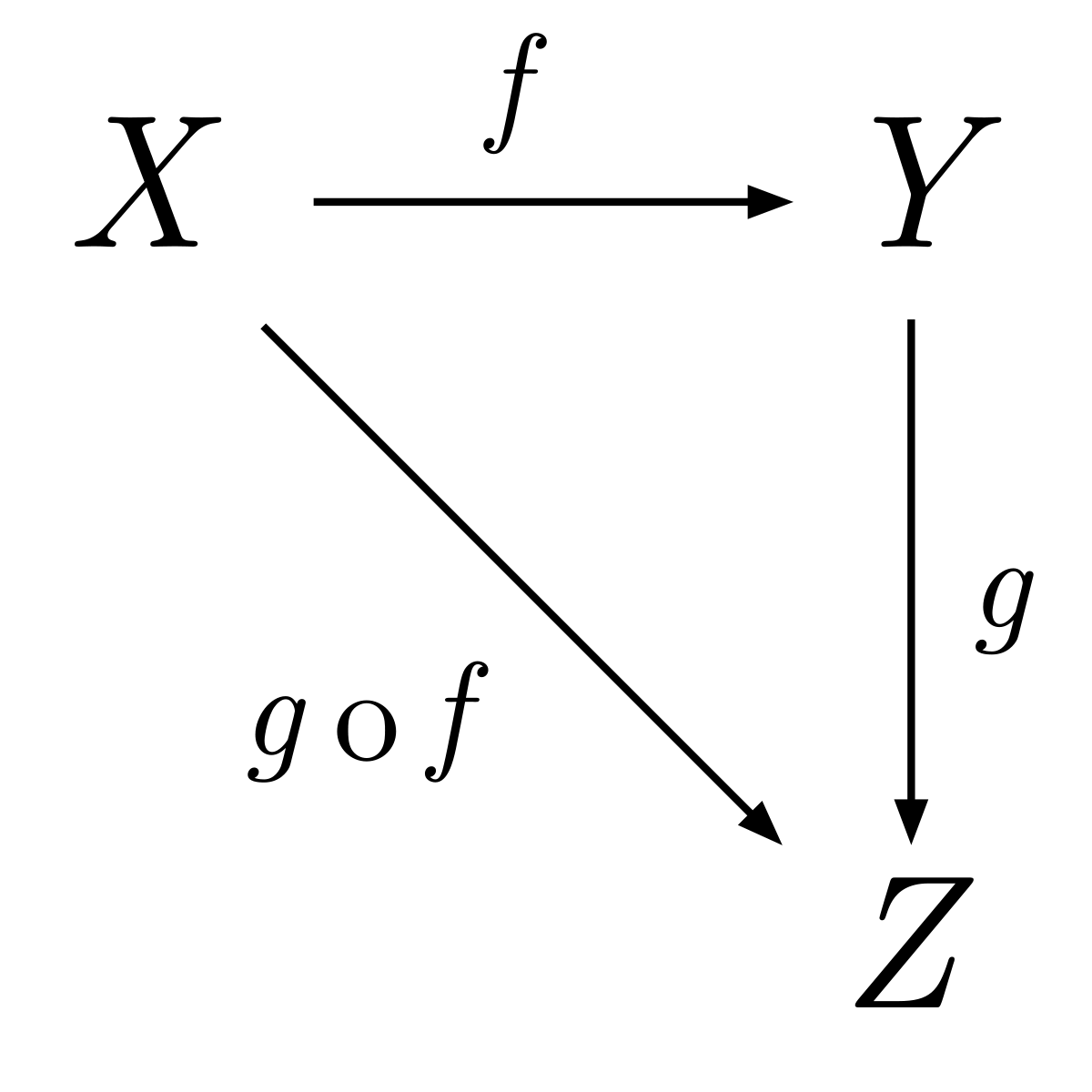We use cookies and other tracking technologies to improve your browsing experience on our site, analyze site traffic, and understand where our audience is coming from. To find out more, please read our privacy policy.

By choosing 'I Accept', you consent to our use of cookies and other tracking technologies.

We use cookies and other tracking technologies to improve your browsing experience on our site, analyze site traffic, and understand where our audience is coming from. To find out more, please read our privacy policy.

By choosing 'I Accept', you consent to our use of cookies and other tracking technologies. Less

We use cookies and other tracking technologies... More

# Login or registerto boost this post!

Show some love to the author of this blog by giving their post some rocket fuel 🚀.

# Login or register to start working on this issue!

Engineers who find a new job through Functional Works average a 15% increase in salary 🚀# Functors and Category Theory Introductory

## Functors and category theory

I think the best way to start learning functors is to look at where they came from. The founders of category theory , Samuel Eilenberg and Saunders Mac Lane (1945) introduced categories as preparation for what they called “functors” and “natural transformations”.

Ah ha !

Then that’s where we’ll start.

This is another one of those topics where you could read a whole book on the subject , but I’m only going to teach you category theory relevant to understanding functors. One resource that I highly recommend that explains category theory is the Stanford Encyclopedia page on it. I’ll be making references to them throughout.

So , what is category theory ?

To put it bluntly , it is the study of categories. A category is a structure comprised of objects and their morphisms.

Note:

## Objects

→ are the elements in a category

## Morphisms

→ are the functions applied on an element that return a new one. Morphisms connect objects to each other and form the category structure.

This is a basic representation of what a category looks like :##### reference: Wikipedia

It was noted in the early days that “objects play a secondary role and could be entirely omitted from definition”. But over time it became accepted to refer to categories using sets of objects, in this case the category C would be defined as

``C =  {X ,  Y,  Z} ``

which in my opinion is much easier to understand.

Given our category C , to be a category , it must satisfy the following conditions:

Morphisms:
For every pair X, Y of objects, there is a set {X, Y}, called the morphisms from X to Y in C. If f is a morphism from X to Y, we write f: X → Y.
This means that there is a function that takes an object X and returns a new object Y. This pairing happens with all objects in the category.

In our category there are the morphisms :

f : X → Y , allowing the pairing {X , Y}
g : Y → Z , allowing the pairing {Y , Z}
g ○ f : X → Z , allowing the pairing {X , Z}

Identity :
For every object X, there exists a morphism idX in {X,X}, called the identity on X. This means there is a function that returns the object it was given . The point of this morphism is to preserve identity. If the function returns something other than the object given, that means the category itself has been altered and hence identity lost.

Therefore we need to add the following identity morphisms into C so we can withhold identity.

idX: X → X , allowing the pairing {X, X}
idY: Y → Y , allowing the pairing {Y, Y}
idZ : Z → Z , allowing the pairing {Z, Z}

Composition :
For every triple X, Y and Z of objects, there exists a composition of morphisms in C. If f : X → Y and g : Y → Z, the composition of f and g is notated (g ○ f ) : X → Z . This rule must hold as otherwise the category wouldn't be an interconnected structure.

Alright , what’s this got to with functors I hear you ask?

We’re almost there , in this next section we’re going to learn what makes a functor a functor . I’m going to provide you another extract from Eilenberg and Mac Lane’s work in which they talk about the role of a functor in category theory:

The idea of a category is required only by the precept that every function should have a definite class as domain and a definite class as range, for the categories are provided as the domains and ranges of functors. Thus one could drop the category concept altogether and adopt an even more intuitive standpoint, in which a functor such as “Hom” is not defined over the category of “all” groups, but for each particular pair of groups which may be given.

That was probably a lot to take in. But don’t despair !

This kind of links back to what was said about objects being a secondary role. The extract states we could drop objects and categories all together. Instead we define a functor called “Hom” and that there is a set of inputs (domain) , lets call X , and a set of outputs (range) , lets call Y, for which they create the mapping {Xi , Yi} for a particular grouping of domain and range.

And there in lies the definition.

A functor is a mapping between categories.

In this case the different categories are omitted as domain and range , but hopefully you get the picture.

OK , I think it’s time for a recap on all this new knowledge.

Categories are structures that are made from objects and morphisms that connect them. Morphisms are functions which takes one object and return a new one . The interconnectivity builds a category which can be used to model real world structures. Functors have the ability to map one category to another , without altering the morphisms between the category itself to do so. Functors satisfy the condition that each object must have it’s own identity.

Quick Q & A time →

### Q:

Is a functor a function ?

### A:

Yes , one that takes a category and maps it to another.

### Q:

A functor is the mapping between structures , is the functor itself mappable ?

### A:

This is where all the confusion and arguments start . What I think will help us here is this section on the composition of functors (pg.20). Basically, a functor does allow another functor as an argument, making it a higher order function but it does not make it mappable. Think of it this way, if we assume that functors are mappable we could associate things like a vector as being a functor, because a vector is mappable. But that would be ridiculous. A functor is the mapping between categories , and I don’t know about you but I haven’t seen any vectors that can map categories.

If we look at the word "functor" it is similar to "operator" and an operator will perform operations on data. This is what a functor does. It takes operands (categories) and links them. To say the functor is mappable would assume the functor is the operand, which isn't the case. A succinct explanation can be found here.

Example :

Let’s define a category C as a linked list

``(2 4 8 16 32) ``

If this were a category we could say the morphism is to double the given element and return the new element.

Now another category D as a linked list

``(4 16 64 256 1024) ``

If this were a category we could say the morphism is to multiply the given element by 4 and return the new element.

Now, what would our functor need to do to map the following categories?

We could write

``(map #(* % %)  C)``

which would be suitable and allow us to map each object in C to each object in D.

But this isn’t perfect . The clojure definition of map returns a list , what if we had used vectors?

So

``C = [ 2 4 8 16 32] ``

and

``D = [4 16 64 256 1024]``

Then our mapping of C would be different to the category D , as we would have had

`` (4 16 64 256 1024)``

which would have been wrong in this case.

The “fmap” functor for mapping would return the type it is given. Then it would be suitable as we always map to D and not some other category of a different type.

But there is another layer to this, look at this example :

What if I wanted to map these two categories?

``````C = (2 4 8 16 32)
D = [4 16 64 256 1024]``````

We can’t use fmap , do you know what we would have to write ?

All we need to do is utilise the vec functor to map categories like C , to categories like D.

``(vec (map #(* % %)  C))``

And that my friends about wraps this article up ! I hope you have enjoyed , here some other pieces on functors and category theory that you may find useful.

References :